[Log-In] or [Register]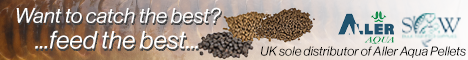Advertise to thousands of anglers a day!  Click HERE to see howNew Posts: 0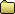Using Glass Spheres for pop ups - CAUTION [Log-In]  [Register]
 tinofmaggots Posts: 5835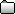#13 25 Oct 2011 at 7.22pm
 In reply to Post #10Volume of a sphere Circumscribed cylinder to a sphere. In 3 dimensions, the volume inside a sphere (that is, the volume of a ball) is given by the formula \!V = \frac{4}{3}\pi r^3 where r is the radius of the sphere and π is the constant pi. This formula was first derived by Archimedes, who showed that the volume of a sphere is 2/3 that of a circumscribed cylinder. (This assertion follows from Cavalieri's principle.) In modern mathematics, this formula can be derived using integral calculus, e.g. disk integration to sum the volumes of an infinite number of circular disks of infinitesimal thickness stacked centered side by side along the x axis from x = 0 where the disk has radius r (i.e. y = r) to x = r where the disk has radius 0 (i.e. y = 0). At any given x, the incremental volume (δV) is given by the product of the cross-sectional area of the disk at x and its thickness (δx): \!\delta V \approx \pi y^2 \cdot \delta x. The total volume is the summation of all incremental volumes: \!V \approx \sum \pi y^2 \cdot \delta x. In the limit as δx approaches zero this becomes: \!V = \int_{-r}^{r} \pi y^2 dx. At any given x, a right-angled triangle connects x, y and r to the origin, hence it follows from Pythagorean theorem that: \!r^2 = x^2 + y^2. Thus, substituting y with a function of x gives: \!V = \int_{-r}^{r} \pi (r^2 - x^2)dx. This can now be evaluated: \!V = \pi \left[r^2x - \frac{x^3}{3} \right]_{-r}^{r} = \pi \left(r^3 - \frac{r^3}{3} \right) - \pi \left(-r^3 + \frac{r^3}{3} \right) = \frac{4}{3}\pi r^3. Therefore the volume of a sphere is: \!V = \frac{4}{3}\pi r^3. Alternatively this formula is found using spherical coordinates, with volume element \mathrm{d}V=r^2\sin\theta\,\mathrm{d}r\,\mathrm{d}\theta\,\mathrm{d}\varphi In higher dimensions, the sphere (or hypersphere) is usually called an n-ball. General recursive formulas exist for deriving the volume of an n-ball. For most practical uses, the volume of a sphere can be approximated as 52.4% of the volume of an inscribing cube, since \pi/6 \approx 0.5236. For example, since a cube with edge length 1 m has a volume of 1 m3 , a sphere with diameter 1 m has a volume of about 0.524 m3 . Thought you knew all that Brian.
 nwpiker41 Posts: 8093#12 25 Oct 2011 at 6.46pm
 In reply to Post #11Based on the evidence thus far, i have put the 2 main posts at the top of the thread.If this all turns out to be factual it can be made a sticky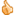. Can we please have our resident bait makers input on this please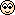.
 silverfish Posts: 2393#11 25 Oct 2011 at 6.32pm
 In reply to Post #10Hollow as in 'glass bubbles'.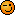what do they look like...........in the bag.......a very fine light powder, out of the bag........a very fine powder that slowly drops to ground level and floats around if disturbed. A bit like powder snow or wading around in polystyrene balls. Buoyancy........think of an airball pop-up as being 20% micro encapsulated air
 Brian_Woolsey Posts: 21632#10 25 Oct 2011 at 5.58pm
 are these spheres solid glass? or hollow??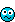how do they make bait so buoyant?? i've never used, nor am i likely to seeing as i hate bait making! these nor have any idea what they look like.
 mattycarphunter Posts: 1276#9 25 Oct 2011 at 5.53pm
 In reply to Post #8Agreed Keith IF, and thats a very big IF, you do follow the precautions, which is my point exactly. Matt
 mattycarphunter Posts: 1276#7 25 Oct 2011 at 11.53am
 In reply to Post #6you might say you would never do it to bait....bet a lot of people think that, and don't realise that half the pop ups they've got are glass!!! The effect on the fish is secondary in my opinion, how many pop ups get ingested by carp - not many! Spread the word to anyone you know that's even thinking about making their own. cheers Matt
 Bungz Posts: 371#6 25 Oct 2011 at 8.19am
 In reply to Post #5are glass spheres any good for fish ? I cant say id ever do this to baitwandle1 Posts: 6989#5 25 Oct 2011 at 0.49am
 In reply to Post #4 Learn something everyday.....didnt know that ...good info..
 mattycarphunter Posts: 1276#4 24 Oct 2011 at 10.56pm
 In reply to Post #3silverfish great link which backs up what i am saying. I am reading more and more "how do i make pop ups" replied with "just add 20% glass spheres..." Be warned. And I ask the Mods again, this really should be a sticky - plenty of stickies about fish safety, this one is even more important, its anglers' safety. For those that dont read silverfish's link,its the health and safety data sheet from the manufacturer 3M and it recomends proper goggles and PROPER facemask, not just a thin dust mask like you would wear decorating or something. Be safe guys! Matt
 silverfish Posts: 2393#3 24 Oct 2011 at 9.59pm
 Russ_C Posts: 3223#2 24 Oct 2011 at 9.51pm
 In reply to Post #1good warning postAdvertising disclosure © Copyright 2002-2023  -  www.CarpForum.co.uk contact : webmaster@carpforum.co.uk